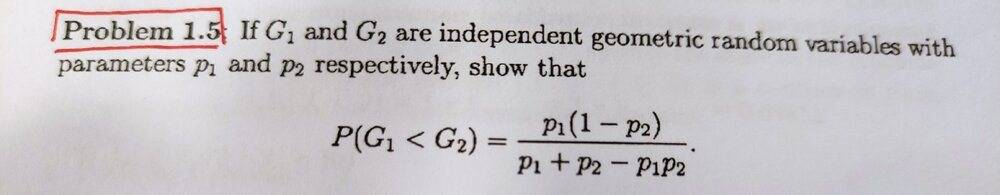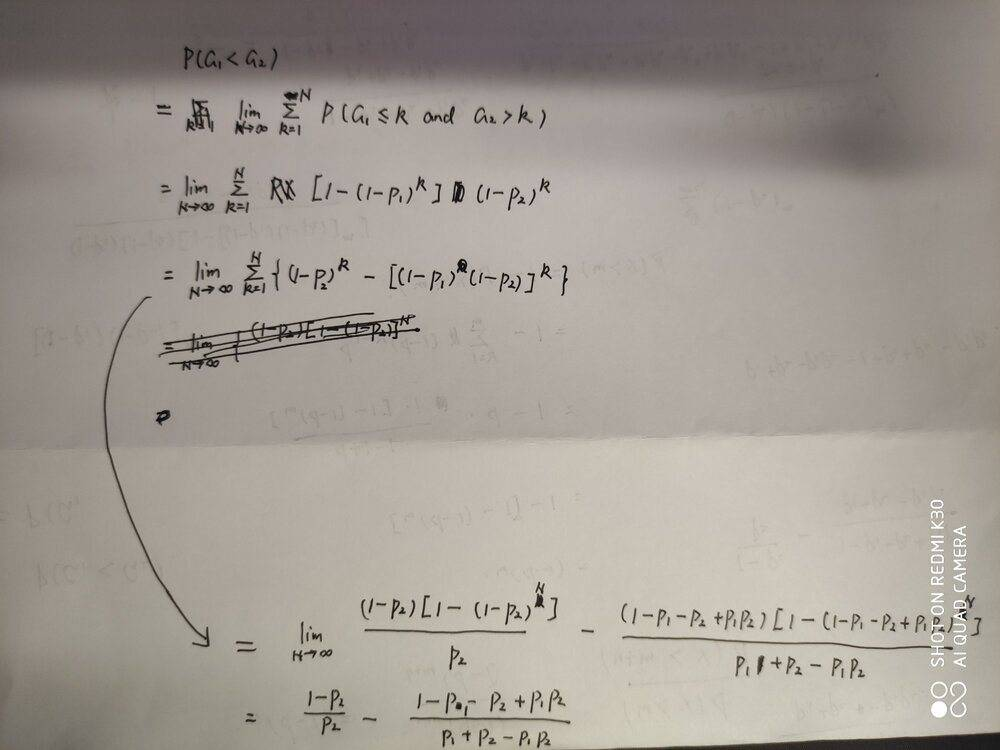# Proof of a formula with two geometric random variables

• Armine

#### Armine

Homework Statement
If G_1 and G_2 are independent geometric random variables with parameters p_1 and p_2 respectively, show that
Relevant Equations
P(G_1<G_2)=p_1(1-p_2)/(p_1+p_2-p_1p_2)
The image above is the problem and the image below is the solution I have tried but failed.•